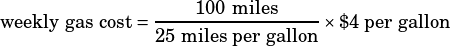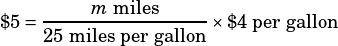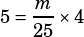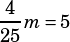Practice test 5, Section 3, Prob 15: How would you think through this problem to get the right answer?

This is a tough one, right? The key is to come up with an equation for how much Alan spends on gas per week. You know he drives 100 miles, gets 25 miles per gallon of gas, and that gas costs $4 per gallon. From that info, we can say that:Simplifying the right side will tell you that he spends$16 per week, but the structure of the equation is what’s important. Once you’ve got that, you can make the adjustment to figure out how many miles (m) he needs to drive to spend $5 (which of course is the same as the number of miles he needs to NOT drive to NOT spend$5):Do a little rearranging and you’ve got answer choice D:Kiran Zaidi says:
how did you know to set the equation equal to 5$?isabellem says: If Alan wants to reduce his weekly expenditure BY$5 dollars, doesn’t it mean he wants to spend $5 less than he normally would? So yeah, why is it equal to$5? not like x-5 for something where x is the original amount he spends (so 16-5=11)Mike McClenathan says:
The important part of the post for your question is this: “Once you’ve got that, you can make the adjustment to figure out how many miles (m) he needs to drive to spend $5 (which of course is the same as the number of miles he needs to NOT drive to NOT spend$5)